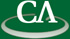## Two fundamental problems in computational linear algebra.

### Michael Monagan, CECM.

Wednesday July 15th, 2009, in K9509 at 3:30pm.

```We consider two fundamental computational problems in linear algebra.
The first problem is how to compute the determinant of a matrix A where the entries
of A are in an integral domain D.  The algorithm I'll present is known as the
Bareiss fraction-free algorithm.  It was independently discovered by Edmonds in 1967
and Bareiss in 1968 although Bareiss states that it was known by Jordan.

The second problem is how to solve the linear system Ax=b for x over the rationals.
Here I'll present two modular methods.  The first combines Chinese remaindering with
Cramer's rule -- yes, Cramer's rule can actually be useful in computation!
The second, the faster method, uses a p-adic lifting approach and
rational number reconstruction that was introduced last time.

Both of these computational problems have a very long history
starting with Gauss and yet are still not completely solved.
```